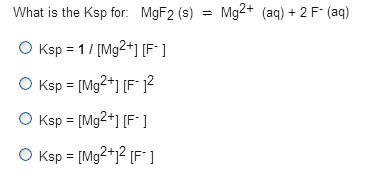# Problem: What is the Ksp for MgF2 (s) = Mg2+ (aq) + 2 F-(aq) a. Ksp = 1/[Mg2+] [F-]b. Ksp = [Mg2+] [F-]2 c. Ksp = [Mg2+] [F-]d. Ksp = [Mg2+]2[F-]

🤓 Based on our data, we think this question is relevant for Professor Chmaissem's class at NIU.

###### FREE Expert Solution###### Problem Details

What is the Ksp for MgF2 (s) = Mg2+ (aq) + 2 F-(aq)

a. Ksp = 1/[Mg2+] [F-]

b. Ksp = [Mg2+] [F-]2

c. Ksp = [Mg2+] [F-]

d. Ksp = [Mg2+]2[F-